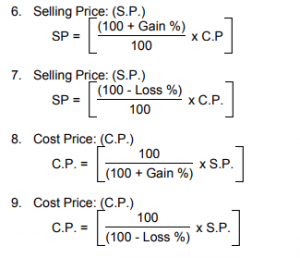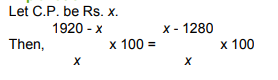## Profit & Loss

### Profit & Loss

Cost Price:
The price, at which an article is purchased, is called its cost price, abbreviated as C.P.
Selling Price:
The price, at which an article is sold, is called its selling prices, abbreviated as S.P.
Profit or Gain:
If S.P. is greater than C.P., the seller is said to have a profit or gain.
Loss:
If S.P. is less than C.P., the seller is said to have incurred a loss.
IMPORTANT FORMULAE
1. Gain = (S.P.) - (C.P.)

2. Loss = (C.P.) - (S.P.)

3. Loss or gain is always reckoned on C.P.

4. Gain Percentage: (Gain %)
Gain % =5. Loss Percentage: (Loss %)
Loss % =10. If an article is sold at a gain of say 35%, then S.P. = 135% of C.P.

11. If an article is sold at a loss of say, 35% then S.P. = 65% of C.P.
12. When a person sells two similar items, one at a gain of say x%, and the other at a loss of x%, then theseller always incurs a loss given by:
Loss % ==13. If a trader professes to sell his goods at cost price, but uses false weights, then
Gain % =1. Alfred buys an old scooter for Rs. 4700 and spends Rs. 800 on its repairs. If he sells the scooter forRs. 5800, his gain percent is:
A.%
B%
C. 10%
D. 12%

Explanation:
Cost Price (C.P.) = Rs. (4700 + 800) = Rs. 5500.
Selling Price (S.P.) = Rs. 5800.
Gain = (S.P.) - (C.P.) = Rs.(5800 - 5500) = Rs. 300.
Gain % ==2. The cost price of 20 articles is the same as the selling price of x articles. If the profit is 25%, then the value of x is:
A. 15
B. 16
C. 18
D. 25

Explanation:
Let C.P. of each article be Re. 1 C.P. of x articles = Rs. x.
S.P. of x articles = Rs. 20.
Profit = Rs. (20 - x).
∴⇒ 2000 - 100x = 25x
125x = 2000
⇒x = 16.

3. If selling price is doubled, the profit triples. Find the profit percent.
A.B. 100

C.D. 120
Explanation:
Let C.P. be Rs. x and S.P. be Rs. y.
Then, 3(y - x) = (2y - x)⇒   y = 2x.
Profit = Rs. (y - x) = Rs. (2x - x) = Rs. x.

Profit % == = 100%

4. In a certain store, the profit is 320% of the cost. If the cost increases by 25% but the selling price remains constant, approximately what percentage of the selling price is the profit?
A. 30%
B. 70%
C. 100%
D. 250%

Explanation:
Let C.P.= Rs. 100. Then, Profit = Rs. 320, S.P. = Rs. 420.
New C.P. = 125% of Rs. 100 = Rs. 125
New S.P. = Rs. 420.
Profit = Rs. (420 - 125) = Rs. 295.

∴Required percentage === 70% (approximately)

5. A vendor bought toffees at 6 for a rupee. How many for a rupee must he sell to gain 20%?
A. 3
B. 4
C. 5
D. 6

Explanation:

C.P. of 6 toffees = Re. 1
S.P. of 6 toffees = 120% of Re. 1 = Rs.For Rs., toffees sold = 6.

For Re. 1, toffees sold == 5.

6. The percentage profit earned by selling an article for Rs. 1920 is equal to the percentage loss incurred by selling the same article for Rs. 1280. At what price should the article be sold to make 25% profit?
A. Rs. 2000
B. Rs. 2200

C. Rs. 2400

Explanation:⇒1920 - x = x - 1280
⇒2x = 3200
⇒x = 1600
∴Required S.P. = 125% of Rs. 1600 = Rs.= Rs 2000.

7. A shopkeeper expects a gain of 22.5% on his cost price. If in a week, his sale was of Rs. 392, what was his profit?
A. Rs. 18.20
B. Rs. 70
C. Rs. 72
D. Rs. 88.25

Explanation:
C.P. = Rs.= Rs.= Rs. 320

∴Profit = Rs. (392 - 320) = Rs. 72.

8. A man buys a cycle for Rs. 1400 and sells it at a loss of 15%. What is the selling price of the cycle?
A. Rs. 1090
B. Rs. 1160
C. Rs. 1190
D. Rs. 1202

Explanation:

S.P. = 85% of Rs. 1400 = Rs.= Rs. 1190

9. Sam purchased 20 dozens of toys at the rate of Rs. 375 per dozen. He sold each one of them at the rate of Rs. 33. What was his percentage profit?

A. 3.5
B. 4.5
C. 5.6
D. 6.5

Explanation:
Cost Price of 1 toy = Rs.= Rs. 31.25
Selling Price of 1 toy = Rs. 33
So, Gain = Rs. (33 - 31.25) = Rs. 1.75
∴Profit % ==% = 5.6%

10. Some articles were bought at 6 articles for Rs. 5 and sold at 5 articles for Rs. 6. Gain percent is:
A. 30%
B. 33%
C. 35%
D. 44%

Explanation:
Suppose, number of articles bought = L.C.M. of 6 and 5 = 30.

C.P. of 30 articles = Rs.= Rs. 25.

S.P. of 30 articles = Rs.= Rs. 36.

Gain % =% = 44%. 25

11. On selling 17 balls at Rs. 720, there is a loss equal to the cost price of 5 balls. The cost price of aball is:
A. Rs. 45
B. Rs. 50
C. Rs. 55
D. Rs. 60

Explanation:
(C.P. of 17 balls) - (S.P. of 17 balls) = (C.P. of 5 balls)
⇒C.P. of 12 balls = S.P. of 17 balls = Rs.720.

⇒C.P. of 1 ball = Rs.= Rs. 60.

12. When a plot is sold for Rs. 18,700, the owner loses 15%. At what price must that plot be sold in order to gain 15%?
A. Rs. 21,000
B. Rs. 22,500
C. Rs. 25,300
D. Rs. 25,800

Explanation:
85 : 18700 = 115 : x
⇒x == 25300.

Hence, S.P. = Rs. 25,300.

13. 100 oranges are bought at the rate of Rs. 350 and sold at the rate of Rs. 48 per dozen. The percentage of profit or loss is:
A.% gain
B. 15% gain
C.% loss
D. 15 % loss

Explanation:
C.P. of 1 orange = Rs.= Rs. 3.50

S.P. of 1 orange = Rs.= Rs. 4

Gain% =%=% =%

14. A shopkeeper sells one transistor for Rs. 840 at a gain of 20% and another for Rs. 960 at a loss of 4%. His total gain or loss percent is:
A.% loss
B.% gain
C.% gain
D. None of these

15. A trader mixes 26 kg of rice at Rs. 20 per kg with 30 kg of rice of other variety at Rs. 36 per kg and sells the mixture at Rs. 30 per kg. His profit percent is:
A. No profit, no loss
B. 5%
C. 8%
D. 10%
E. None of these

∴Gain =% = 5%.